AP Calculus Multiple Choice Practice Problems

The best way to study for the AP Calculus Exam, whether that is Calculus AB or Calculus BC, is to do practice problems. They will give you an idea of the types of problems that you may encounter, reinforce what you already know, and learn how to approach problems that you have never seen before. The following is a handful of AP Style Multiple Choice Practice Problems (for Calc AB), with the full solutions given. This is certainly not an exhaustive list of all the topics and types of questions, but just some extra AP Calculus multiple choice practice problems that can be used along with your textbook, AP Calculus review books, and old AP Exams.

For the following practice problems, Calculators are Not Permitted.

Practice Problems

1.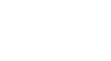What is f(g(2))?

a) -12
b) -8
c) -0
d) 8
e) 12

2. Calculate the following integral: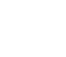a) -1/9
b) -1/27
c) 0
d) 1/27
e) 4/ 9

3. Find the slope of the line tangent to the function f(x) at x = 2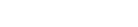a) 17/16
b) 9/8
c) -9/8
d) 2
e) 1

4.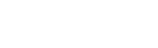a) ∞
b) -∞
c) 0
d) -1/2
e) 1/2

5. Compute the definite integral below: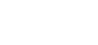a) -/2
b) 0
c) /4
d) /2
e)

6. Find dy/dx for f(x):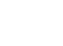a)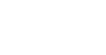b)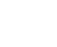c)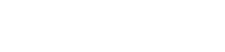d)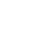e)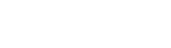7.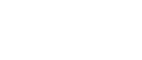a) 0
b) -1/5
c) 0
d) 1/5
e) ∞

8. What is the area bounded between the graphs of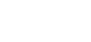a) -4
b) 0
c) -32/3
d) 32/3
e) 32

9.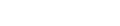Find dy/dx

a)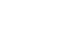b)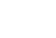c)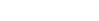d)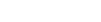e)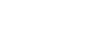10. Where does the following function have a local maximum?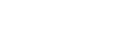a) x = -3
b) x = 0
c) x = 3
d) x = 9
e) x = 27

Solutions

1.First, we evaluate g(2) to get -2/(4+1) = -2/5
Plugging this into f(x), we can evaluate f(-2/5) = 5*(-2/5)-10 = -12

2.This is a fairly straightforward integral that can be solved using the power rule. It’s easier to think it as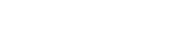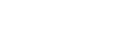3. Anytime we need to find the slope, our first thought should be ‘find the derivative of the function.’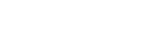Plug in x = 2 to find the slope: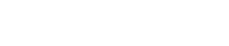4. The solution to this question does not involve any calculation. Anytime we are asked to evaluate a limit as x approaches infinity, look at the degree of the top and bottom equations. If they’re different, our solution is already given. Since in this case, the denominator has a larger degree equation, the limit approaches 0.

5. This is one of our common derivatives/integrals which we should know.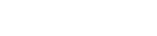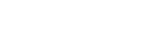Answer: c)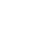6. This is the quotient rule: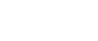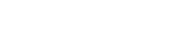in our case: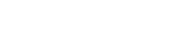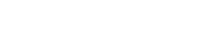Answer: c)(This can also be reduced to a simpler form.)

7. In this case, the denominator and numerator both evaluate to 0. However, if we factor the top and bottom, we notice that a term cancels out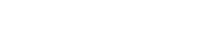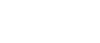8. When we see ‘area bounded by,’ we need to take a definite integral between the points where the graphs intersect. In this case, in since the second equation is y = 0, our limits are the roots of our first equation, x = -2, or x = 2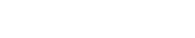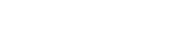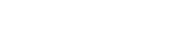9. This is an implicit differentiation problem.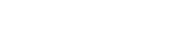Solving for dy/dx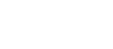we can reduce this to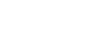Answer: b)10. Anytime we are looking for a local maximum, our first step is to take a look at the derivate.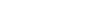Solving for the root of this, we get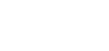The equation has a min and a max at x = 3, and x = -3. You know the max is at x = -3, because if we plug x = -4 into the equation for f’(x), we get a positive answer, so we know the function is increasing before that point.

Improve your SAT or ACT score, guaranteed. Start your 1 Week Free Trial of Magoosh SAT Prep or your 1 Week Free Trial of Magoosh ACT Prep today!Author

•Zachary is a former mechanical engineer and current high school physics, math, and computer science teacher. He graduated from McGill University in 2011 and spent time in the automotive industry in Detroit before moving into education. He has been teaching and tutoring for the past five years, but you can also find him adventuring, reading, rock climbing, and traveling whenever the opportunity arises.